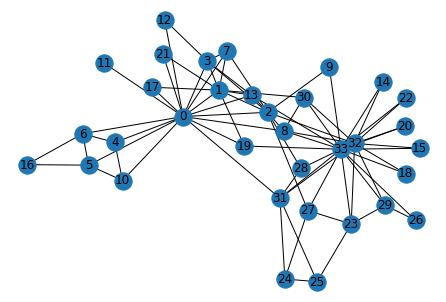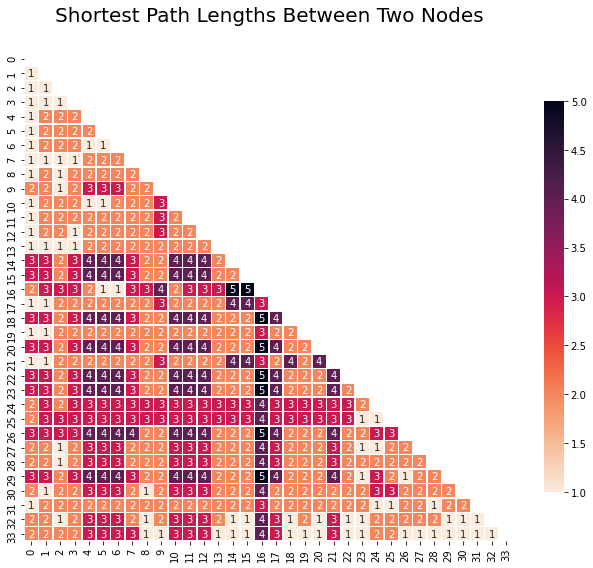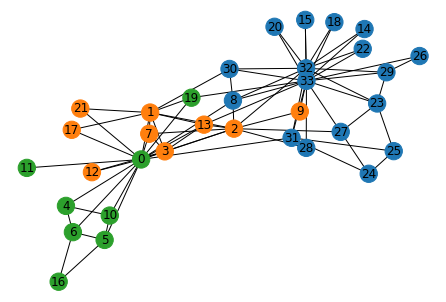Back to Templates

# Network Analysis

Network analysis has a large variety of applications you can try your hand at including social structure analysis, fraud detection and more. In this playbook you will analyze the structure of graphs using the NetworkX package. You will use the popular Zachary's karateclub dataset (source). In the first step of this template, you will load the data, which is in a matrix format, into a NetworkX graph. Afterwards, you will explore the network and derive insights from it in step 2 and 3. Finally, you will visualize these insights in step 4.

```.css-y3yaiy{border:0;border-radius:4px;color:rgb(5, 25, 45);font-family:monospace;font-family:JetBrainsMonoNL;font-size:14px;font-variant-ligatures:none;letter-spacing:0;line-height:1.5;margin:0;padding:0;}```# Load packages
import matplotlib.pyplot as plt
import numpy as np
import networkx as nx
import pandas as pd
import seaborn as sns
color = sns.color_palette()``````

The data should be in matrix format. E.g:

where every element is seperated by a comma in a csv file.

This network is symmetric and would indicate that person 1 and 2 are connected, so are person 1 and 5, and so on.

We will load the very well-known Zachary's karateclub dataset. This dataset is also symmetric, which means that if person 1 is connected to person 2, person 2 is also connected to person 1.

``````# Load data into numpy matrix
FILENAME = "zachary_karateclub.csv"

# Show matrix to check if it is correctly loaded
# A black dot indicates that there is a connection between the two persons
_=plt.imshow(data, cmap='gray_r', aspect='equal')``````### Load data into networkx graph

``````# Set up networkx graph G from numpy matrix
G = nx.from_numpy_matrix(data)``````

## 2. Explore the network

Now that the data has been loaded in a graph correctly, we can start exploring it.

### Number of edges and nodes in graph

``````print(f'The number of edges in the graph: {len(G.nodes())}')
print(f'The number of nodes in the graph: {len(G.edges())}')``````
``````The number of edges in the graph: 34
The number of nodes in the graph: 78``````

### List of neighbors for any node

``````# Node to determine the neighbors for
node = 2
print(f'The neighbors of node {node} are {list(G.neighbors(node))}')

# You can also print it for every node
#for node in G.nodes():
#    print(f'The neighbors of node {node} are {list(G.neighbors(node))}')``````
``The neighbors of node 2 are [0, 1, 3, 7, 8, 9, 13, 27, 28, 32]``

### Show a basic visualization of the graph

``````# Show basic visualization of graph
nx.draw(G, with_labels=True)``````## 3. Derive insights from the graph

### Shortest path between two specific nodes

``````START_NODE = 29
END_NODE = 16

shortest_path = nx.shortest_path(G, START_NODE, END_NODE)

print(f'The shortest path from node {START_NODE} to node {END_NODE} is: {shortest_path}')``````
``The shortest path from node 29 to node 16 is: [29, 32, 2, 0, 5, 16]``

### Calculate degree centrality

``````node = 0

deg_cent = nx.degree_centrality(G)
print(f'The degree centrality for node {node} is {deg_cent[node]:.2f}')

# You can also print it for every node
#for node in G.nodes():
#    print(f'The degree centrality for node {node} is {deg_cent[node]:.2f}')``````
``The degree centrality for node 0 is 0.48``

### Determine the communities

``````# Import the community package from networkx
import networkx.algorithms.community as nxcom``````
``````communities = sorted(nxcom.greedy_modularity_communities(G), key=len, reverse=True)

for i, com in enumerate(communities):
print(f'Community {i}: {list(com)}')``````
``````Community 0: [32, 33, 8, 14, 15, 18, 20, 22, 23, 24, 25, 26, 27, 28, 29, 30, 31]
Community 1: [1, 2, 3, 7, 9, 12, 13, 17, 21]
Community 2: [0, 4, 5, 6, 10, 11, 16, 19]``````

## 4. Visualize insights from graph

### Highlight shortest path betwoon two specific nodes

``````from itertools import combinations

def convert_nodes_to_edges(nodes_list):
edges_list = []
for i, n in enumerate(nodes_list):
if(i<len(nodes_list)-1):
next_n = nodes_list[i+1]
edges_list.append((n, next_n))
return edges_list

START_NODE = 26
END_NODE = 25

shortest_path = nx.shortest_path(G, START_NODE, END_NODE)

pos = nx.spring_layout(G, seed=7)
nx.draw(G, pos=pos, with_labels=True)

shortest_path_edge_list = convert_nodes_to_edges(shortest_path)

_=nx.draw_networkx_edges(G, pos, edgelist=shortest_path_edge_list, width=3, edge_color='red')
``````### Shortest path lengths between nodes

``````b = nx.shortest_path_length(G)
#shortest_paths_df = pd.DataFrame()
shortest_paths_dict = {}
for spl in b:
shortest_paths_dict[spl] = spl

shortest_paths_df = pd.DataFrame.from_dict(shortest_paths_dict)
shortest_paths_df.sort_index(inplace=True)

# Generate a mask for the upper triangle

# Set up the matplotlib figure
fig, ax = plt.subplots(figsize=(11, 9))                    # Set figure size

# Generate a custom diverging colormap
cmap=sns.color_palette("rocket_r", as_cmap=True)

sns.heatmap(shortest_paths_df,
cmap = cmap,
square = True,                                 # Ensure perfect squares
linewidths = 0.5,                              # Set linewidth between squares
cbar_kws = {"shrink": .8},                     # Set size of color bar
annot = True                                   # Include values within squares
);

plt.xticks(rotation=90)                                    # Rotate x labels
plt.title('Shortest Path Lengths Between Two Nodes', size=20, y=1.05);   # Set plot title and position``````### Visualize communities within graph

``````# Import the community package from networkx (if it wasn't imported before)
import networkx.algorithms.community as nxcom``````
``````COLORS = ['tab:blue', 'tab:orange', 'tab:green', 'tab:red', 'tab:purple',
'tab:brown', 'tab:pink', 'tab:gray', 'tab:olive', 'tab:cyan']

def get_index(node, node_groups):
i = 0
while(i<len(node_groups)):
if node in node_groups[i]:
return i
else:
i+=1
return -1

communities = sorted(nxcom.greedy_modularity_communities(G), key=len, reverse=True)

color_map = []

for node in G:
index = get_index(node, communities)
if(index >= len(COLORS)):
print("More groups than colors; add some more colors.")
break
if(index == -1):
print("Node note in a community")
break
color_map.append(COLORS[index])

nx.draw(G, node_color=color_map, with_labels=True)
plt.show()``````### Acknowledgement

There is a lot more you can do with networks. Visit the NetworkX documentation for inspiration.

### Network Analysis

Perform network analysis using the NetworkX package.

Use Template